# Count number of new lines in a cell

In this article, we will learn how to count the line break in cell in Excel.

If a cell contains multiple lines and you need to count the number of lines then you will need to count the line break within cell. A new line is added within a cell using line break character. It is non printable but the character code is 10 (CHAR(10)).

So to count the line breaks, we will use a formula having LEN & SUBSTITUTE function.

The LEN function in Excel counts characters in a string. I mainly use it when I need to extract text from string.

SUBSTITUTE function finds and replaces the new text with old text in the text string.

Now we will make a formula out of it.

1. Firstly, we will count the total number of characters in a cell.
2. Secondly, we will find and replace the line break character ( code = 10 ) with null value.
3. Now we will count the characters of the new string and take a difference from the first count.
4. Now we got the count of number of line breaks, so we add plus 1 to it, to get the count of lines in a cell.

Syntax:

= LEN ( string ) - LEN (SUBSTITUTE( string , CHAR (10), "" )) + 1

Let’s understand this via testing it on some examples.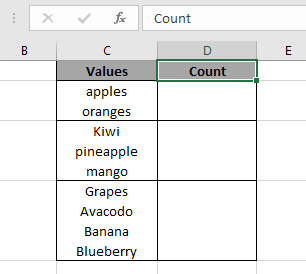Here we have names of fruit as values. And we need to count the number of fruits in the database using Excel formulas.

Use the formula

=LEN ( C2 ) - LEN ( SUBSTITUTE ( C2 , CHAR (10) , "" ) )+1

Explanation:
LEN ( C2 ) will return 14.
LEN(SUBSTITUTE ( C2 ,CHAR (10) ,"" )) will return 13.
+1 to get the count of lines in a cell.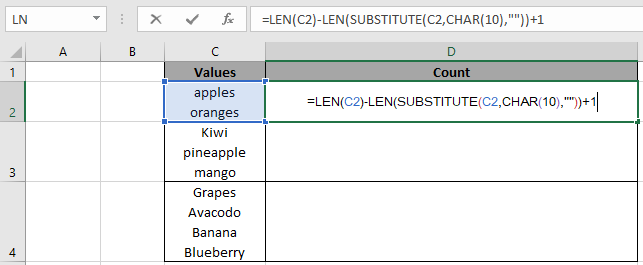Here the arguments to the function is given as cell reference. Press Enter to get the results.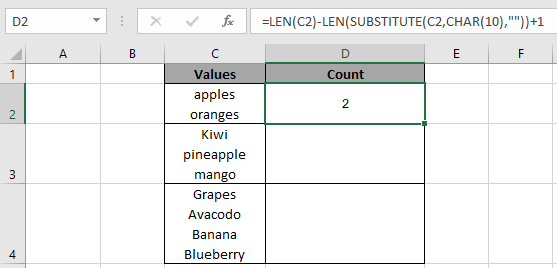Here the function returns 2 as the number of fruits in C2 cell.
Copy the formula in other cells, select the cells taking the first cell where the formula is already applied, use shortcut key Ctrl + D.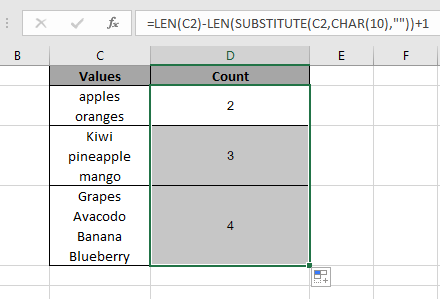Now we got the count of number of different values in the respective cells.

Now get the count of all values via summing up all the count values.
Use the formula:

= SUM ( D2 : D4 )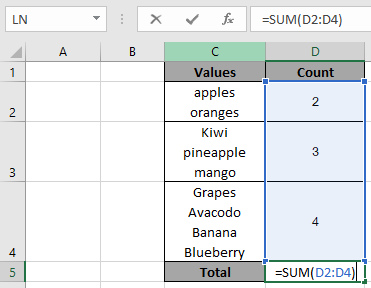The argument values to the function is given as cell reference.
Press Enter to get the total count.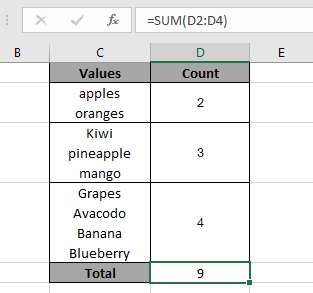As you can see the function returns 9, count of all the fruit names.

Note :
The function returns the count 1 for the blank cell, So to ignore blank cells use the below formula.

Use the formula:

=IF(ISBLANK(B5), 0, LEN(B5)-LEN(SUBSTITUTE(B5,CHAR(10),""))+1)

The above formula catches the blank cell or it returns the outcome for all other values.

Hope you understood how to count the number of lines in Excel. Explore more text function article here. Please feel free to state your queries in the comment box. We will certainly help you.

Related Articles

Count Characters in a Cell in Excel

Excel CHAR(10) Not Working. How to Solve It?

How to use the LEN function in Excel

How to use the SUBSTITUTE function in Excel

Excel REPLACE vs SUBSTITUTE function

Remove unwanted characters in Excel

Popular articles

Edit a dropdown list

If with conditional formatting

If with wildcards

Vlookup by date

Terms and Conditions of use

The applications/code on this site are distributed as is and without warranties or liability. In no event shall the owner of the copyrights, or the authors of the applications/code be liable for any loss of profit, any problems or any damage resulting from the use or evaluation of the applications/code.